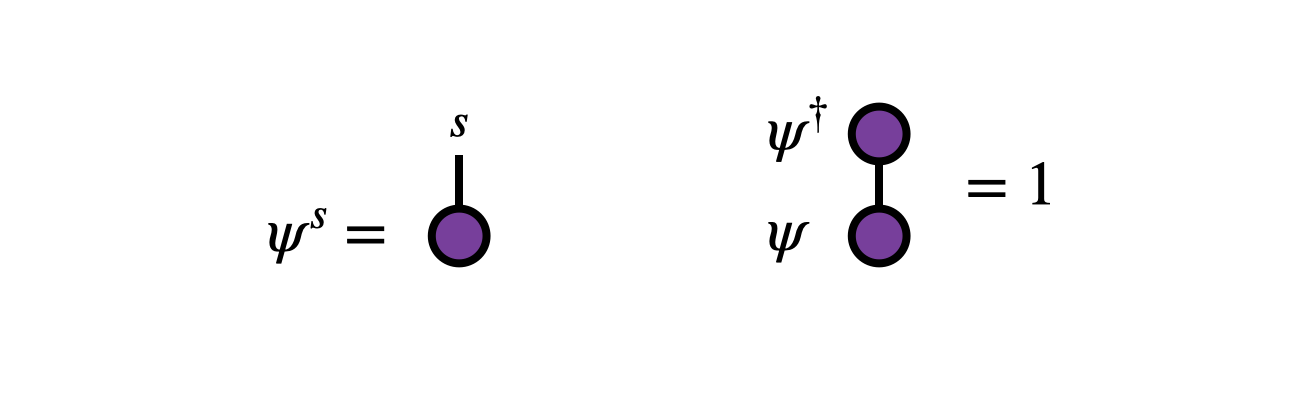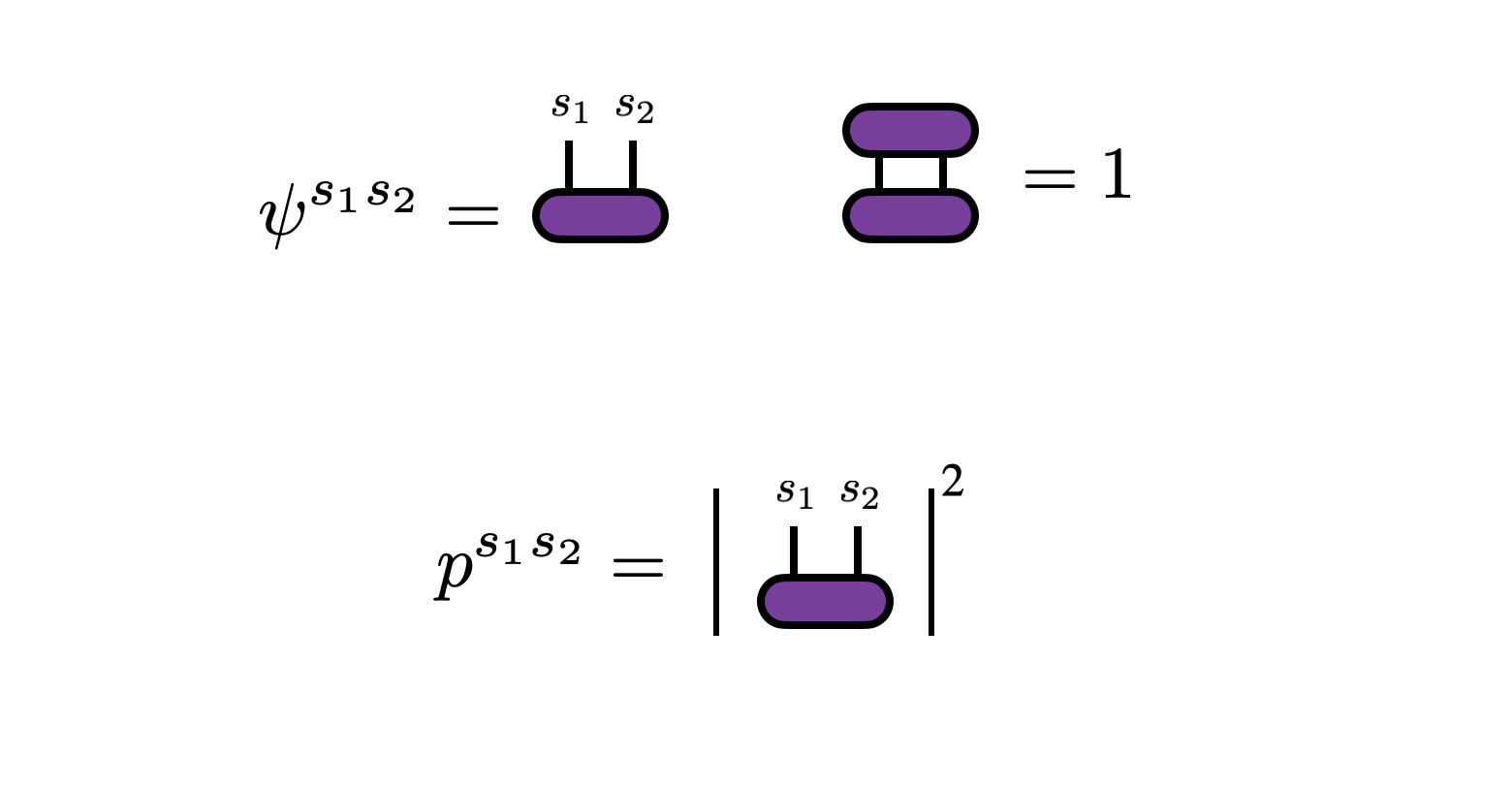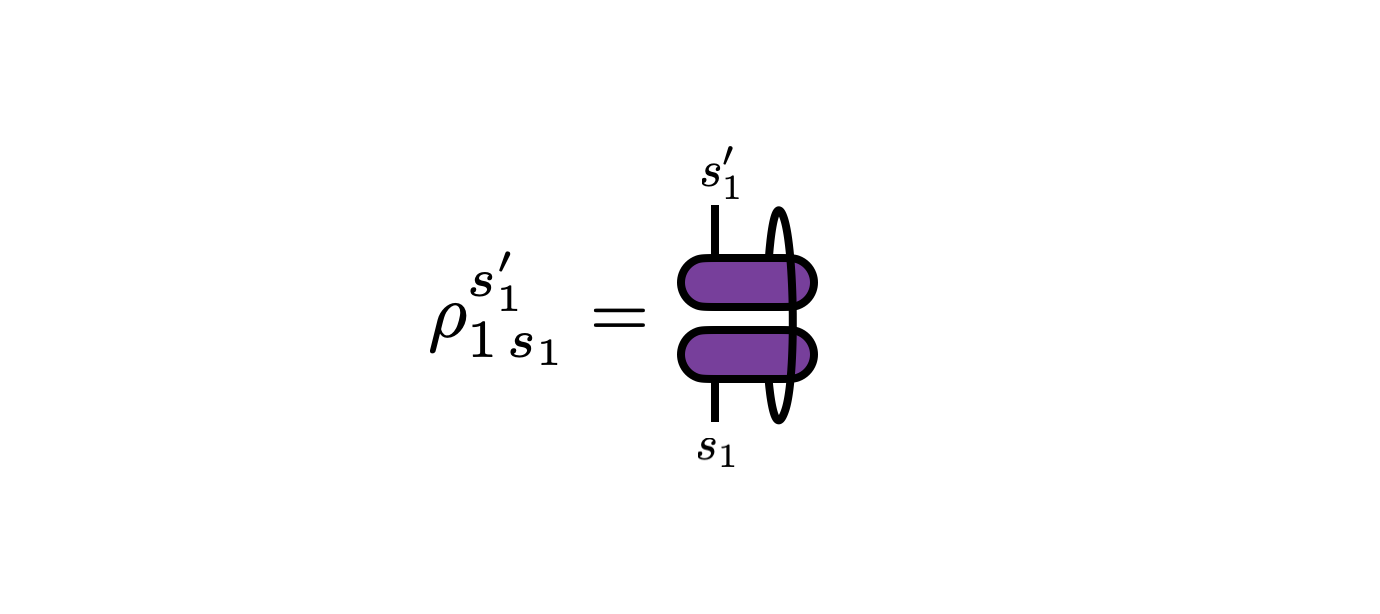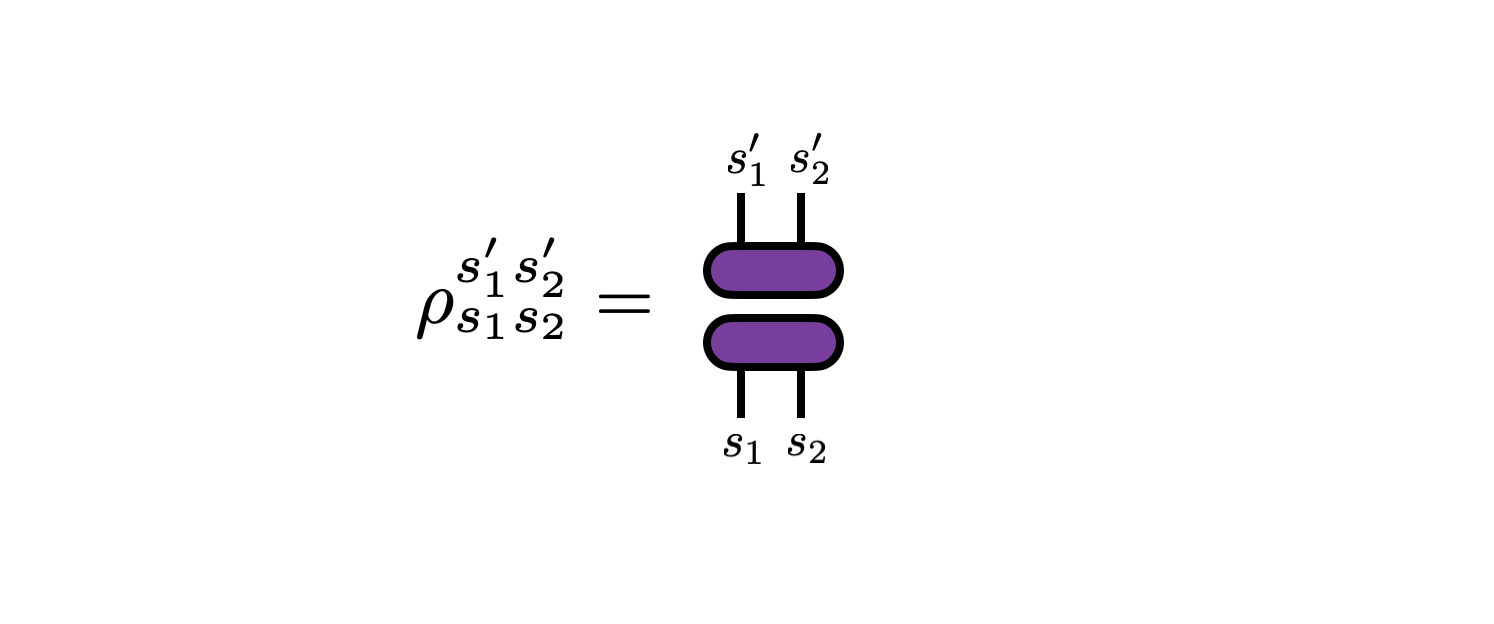About Contribute Source main/statistics/2norm_probability/

Two-Norm or Born Rule Probability Formalism

In addition to the standard probability formalism, where probabilities are positive numbers which add up to one, there is a lesser-known but practical and elegant “2-norm” formalism based on complex numbers whose squares add up to one. This formalism is used extensively in quantum mechanics (where it is known as the Born rule), to the point where the two formalisms are often identified. However, 2-norm probability can be viewed as a purely mathematical formalism with certain appealing aspects compared to standard or “1-norm” probability.

For simplicity, we will only consider discrete probability distributions—the generalization to continuous distributions is done in a similar fashion as in standard probability theory.

(Technical note: physicists often use the word “state” as a synonym for “distribution”. Here we will just use the term “distribution”.)

Basic Formalism

Consider a random variable $s$ taking one of $d$ values, that is $s=1,2,...,d$. To parameterize the probabilities of each of these outcomes, one associates numbers $\psi^s$ called amplitudes which can be positive, negative, or even complex.

The only requirement of these amplitudes is that their squared magnitudes sum to one: @@ \sum_{s=1}^d |\psi^s|^2 = 1 @@ Viewed as a vector $\vec{\psi}$, the amplitudes have unit norm under the Euclidean or 2-norm:From the amplitudes, one defines the probability of observing an outcome $j$ as @@ p^s = |\psi^s|^2 @@The standard 1-norm formalism applies to the probabilities $p^s$, thus one can think of standard probability as fitting “on top” or as a corollary of 2-norm probability theory. We will see below that standard probability also relates to 2-norm probability in other interesting ways.

The most general linear maps taking a valid 2-norm distribution into another valid distribution are unitary transformations—matrices $U$ for which $U U^\dagger = 1$. This follows from the fact that unitary transformations preserve the 2-norms of complex vectors. In contrast, the linear maps which preserve valid 1-norm (or standard) probabilities are stochastic matrices, which are a more limited and less convenient class of matrices compared to unitary matrices. (For example, the very useful singular value decomposition results in factor matrices which are unitary.)

Marginals and Densities

When considering probability distributions whose outcomes are labeled by two or more variables (so called “joint distributions”) one can introduce the concept of marginal distributions where only a subset of outcomes are considered or observed. In 2-norm probability theory, marginal probabilities naturally give rise to the concept of “density matrices” which we will here just call densities for short.

For simplicity, consider a 2-norm probability distribution $\psi^{s_1 s_2}$ over a two-dimensional space of outcomes $(s_1, s_2) = (1,1), (2,1), (1,2), (2,2), ..., (d,d)$. By definition, the probabilities of observing each outcome is given by $p^{s_1 s_2} = |\psi^{s_1 s_2}|^2$.Thus, following standard probability theory, the marginal probabilities $p^{s_1}$—that is, the probabilities of observing outcomes $s_1$ without observing $s_2$—are given by summing over $s_2$: @@ p^{s_1} = \sum_{s_2} p^{s_1 s_2} = \sum_{s_2} |\psi^{s_1 s_2}|^2 = \sum_{s_2} \psi^{s_1 s_2} \psi^\dagger_{s_2 s_1} @@In the last expression above, $p^{s_1}$ is suggestively written as the diagonal of a matrix formed by multiplying the $d\times d$ matrices $\psi$ and $\psi^\dagger$ together. It turns out to be interesting to extend $p^{s_1}$ into an entire matrix by including the off-diagonal elements too, where this matrix @@ \rho_{1\, s_1}^{s'_1} = \sum_{s_2} \psi^{s^\prime_1 s_2} \psi^{\dagger}_{s_2 s_1} @@ is known as the “reduced density matrix” for $s_1$ or just the “reduced density” over $s_1$.By construction, the diagonal entries of this matrix are the marginal probabilities $p^{s_1} = \rho_{1\, s_1}^{s_1}$. The off-diagonal entries of $\rho_1$ take more care to interpret: among other things, they capture information about how much $s_1$ is correlated with the other dimensions that were marginalized over (in this case just $s_2$).

Note that from it follows from its definition that $\rho_1$ is a Hermitian, positive-semi-definite matrix whose trace is equal to 1. We can actually take these properties as the definition of a density.

There is a density $\rho$ we can form out of $\psi^{s_1 s_2}$ whose diagonal elements are the joint distribution over both $s_1$ and $s_2$. This density is given by the outer product of $\psi$ with itself:Densities which have rank 1, such as this one, are known as “pure”. Otherwise a density is called “mixed”.

Mixing Densities

Two-norm probability admits an interesting extension, used often in quantum mechanics applications, where standard (1-norm) probability can be “layered on top”. We have already seen the main ingredients of this formalism: density matrices whose diagonal elements have the interpretation of probabilities.

Densities are formed by taking tensor products of amplitudes and their conjugates and possibly tracing (marginalizing) over a subset of the variables. Densities can also be added together. As long as the coefficients are positive and sum to one, the result will be another valid density (this follows from properties of trace-one, positive-semi-definite matrices). Therefore, these coefficients are nothing but probabilities.

Consider two densities $\rho_A$ and $\rho_B$. Another valid density can be formed by taking positive numbers $\alpha_1, \alpha_2$ such that $\alpha_1 + \alpha_2 = 1$. Then @@ \rho_C = \alpha_1 \rho_A + \alpha_2 \rho_B @@ is another valid density. It has the interpretation of a mixture of distributions, where the outcome is described by the distribution $\rho_A$ with probability $\alpha_1$, or by the distribution $\rho_B$ with probability $\alpha_2$.

Forming a mixture of different densities by summing them will result in a density that has a rank larger than one. This is why such densities are called “mixed”.

Though both the standard (1-norm) and 2-norm probability formalisms may seem like just two different choices, or the 2-norm formalism slightly more cumbersome perhaps, there are intriguing tradeoffs that result from choosing one versus the other.

Advantages of the 2-norm probability formalism:

• Conserved under unitary transformations (versus only stochastic transformations for 1-norm). Unitary transformations can be convenient for both analytical and numerical computations. For example, unitaries naturally arise from matrix factorizations like SVD and QR.
• Geometrically, the set of vectors which are admissible 2-norm amplitudes form a smooth sphere, versus in standard probability theory where normalized vectors form a multi-dimensional diamond which has flat sides and sharp corners.
• Amplitude parameters can be negative or even complex valued. This can offer flexibility for numerical computations. In contrast, the requirement of positivity for standard, 1-norm probabilities can be a serious numerical difficulty.
• Because marginal probabilities are computing by squaring and contracting, or tracing, part of a tensor or tensor network, and because of the relationship to the unitary group, certain tensor networks such as matrix product states can be brought into “canonical forms” where many of the tensors cancel out of certain computations.
• Densities (2-norm analogues of marginals) contain off-diagonal elements which encode information about correlations with marginalized variables.

Drawbacks of the 2-norm probability formalism:

• Because tensors occur twice in computations of probabilities or marginals, there can be a higher computational cost for computing certain quantities compared to standard, 1-norm probability.
• Certain quantities like individual elements of densities can be hard to interpret. Extra quantities and concepts can occur which may not be welcome or necessary for many purposes.

References

1. Phys 771: Lecture 9, Quantum, Scott Aaronson (2006)